## An Alternative Traceback Method for Nussinov's RNA Folding Algorithm

Nussinov's algorithm takes a given sequence of RNA and determines the the most stable secondary structure for that sequence based on the assumption that the more base pairs a structure has, the more stable the structure will be. The algorithm can be divided into two steps:

• First, a matrix M is created such that any cell Mij represents the maximum number of base pairs that a structure consisting of bases i through j could have. The matrix is filled incrementally by sweeping diagonally and filling each upper diagonal using values from the previous diagonal. That is, each substructure is scored and extended from a single base to the entire sequence so that the score of the optimal structure for the entire sequence is in the final cell in the upper right hand corner of the matrix.
• Second, it is necessary to determine which bases are paired in this structure, which requires going backwards from the upper right corner through the matrix and recording which bases are paired. An important detail is that for any given sequence, there are often multiple structures that are considered to be optimal by the algorithm.

Though there are various enhancements that can be made to bring the algorithm more in line with actual structures by modifying how the substructures are scored, the focus of this report is on how an alternate traceback algorithm can be used to obtain better results from Nussinov's algorithm that are normally discarded. Specifically, the new algorithm incorporates the following assumptions:

• Longer stems (consecutive base pairs) are more stable than shorter stems
• A single loop or bulge is more stable than one split in two by a base pair in the middle

Generally speaking, the algorithm attempts to choose a traceback path that will extend opened loops and extend stems when possible while still acting within the constraints of the original algorithm. In pseudocode, the new traceback algorithm can be described as:

```last_direction = NONE
while tb_stack is not empty:
pop(tb_stack, i,j)
if i >= j:
continue
if γ(i+1,j-1) + δi,j == γ(i,j):
push(candidate_stack, DIAGONAL, i+1,j-1)
if γ(i,j-1) == γ(i,j):
push(candidate_stack, LEFT, i,j-1)
if γ(i+1,j) == γ(i,j):
push(candidate_stack, DOWN, i+1,j)
if candidate_stack is empty:
last_direction = NONE
for k from i < k < j:
if γ(i,k) + γ(k+1,j) == γ(i,j):
push(tb_stack, k+1,j)
push(tb_stack, i,k)
break
else:
while candidate_stack is not empty:
pop(candidate_stack, d, m,n)
if d == last_direction:
push(tb_stack, m,n)
if d == DIAGONAL:
record(i,j)
break
else if nothing pushed onto tb_stack:
push(tb_stack, m,n)
```
 In the canonical implementation of the traceback step, whenever there are multiple structures that are equivalent in terms of number of base-pairs the first structure that works is chosen because the algorithm does not care about anything besides the number of base-pairs, so any structure with the same number of base pairs as the optimal one will do. However, this ignores important information that can lead it to choose an unstable structure over a more stable one. Below in figure 2 are three possible structures as predicted by Nussinov's algorithm. All three meet the constraints of the algorithm and have the maximal three base pairs. However, these structures are not equally stable. Figure 2a is decidedly the most stable of the three. It forms a three base pair long stem and a three base hairpin loop (which is more stable than a shorter hairpin loop because the angle is less sharp). Figure 2b is rather unstable and improbable because it requires two adjacent bases to be paired, which would require bending the bases in a nonsensical way. Figure 2c is not particularly stable, but is reasonable; it is nearly identical to the structure in figure 2a, except that it introduces a small bulge loop into the stem, which would make it less stable.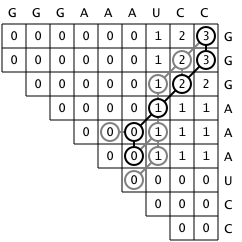Figure 1: This matrix shows a completed traceback. The actual traceback is shown in black while possible paths that were ignored are shown in grey.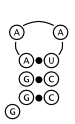Figure 2a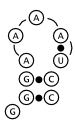Figure 2b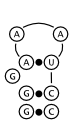Figure 2c
 As noted on this web page, the structure and traceback in figure 10.9 of Biological Sequence Analysis (reproduced here as figure 1 and figure 2a respectively) are inconsistent with the pseudocode description of the standard traceback algorithm given. The structure is arguably the most stable of the possible foldings that Nussinov's algorithm can predict, but is different from the one that the traceback algorithm described in the book would choose, which is shown above in figure 2b.. This alternate version of the traceback algorithm yields the correct traceback corresponding to the optimal structure in figure 2a.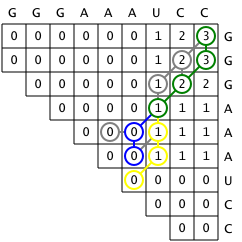Figure 3: The path taken by the standard traceback is shown in yellow, the alternate traceback in blue, and where they overlap in green.

### References

1. Durbin R, Eddy S, Krogh A, Mitchinson G. Biological Sequence Analysis. New York: Cambridge University Press. 1998.
2. Wikipedia contributors. RNA structure. Wikipedia, The Free Encyclopedia. May 9, 2008, 06:22 UTC. Available at: http://en.wikipedia.org/w/index.php?title=RNA_structure&oldid=211205665. Accessed August 2, 2008
3. Sonderegger B. Short Discussion of an Error on Page 271. Bernhard Sonderegger's Masters Program Page. Available at: http://ludwig-sun2.unil.ch/~bsondere/nussinov/Correction.htm. Accessed September 13th, 2006.